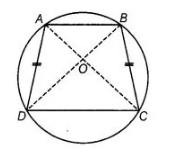# If a pair of opposite sides of a cyclic quadrilateral are equal,`
Question:

If a pair of opposite sides of a cyclic quadrilateral are equal, then prove that its diagonals are also equal.

Thinking Process

Firstly, prove that ΔACD is congruent to ΔBDC by SAS and then further prove the required result

Solution:Join AC and BD.

To prove AC = BD

Proof In ΔAOD and ΔBOC,

∠OAD = ∠OBC and ∠ODA = ∠OCB

[since, same segments subtends equal angle to the circle]

AB = BC [given]

ΔAOD = ΔBOC [by ASA congruence rule]

Adding is DOC on both sides, we get

ΔAOD+ ΔDOC ≅ ΔBOC + ΔDOC